Check Full Chapter Explained - Continuity and Differentiability - Continuity and Differentiability Class 12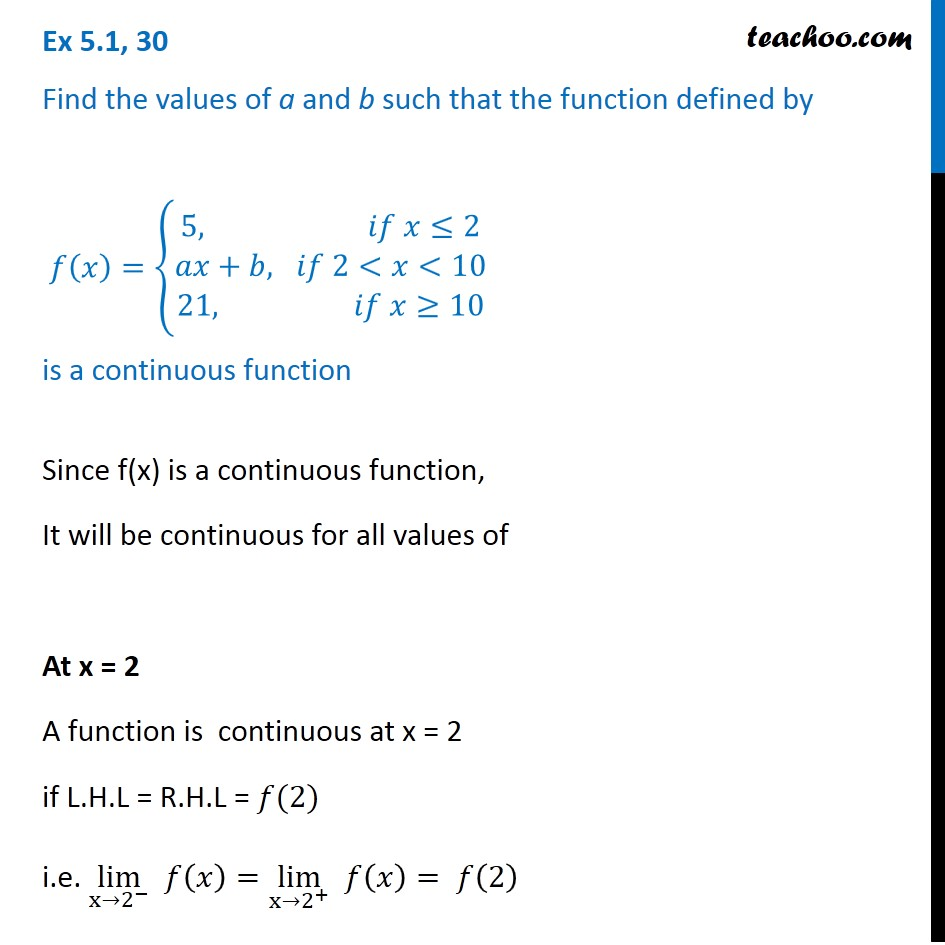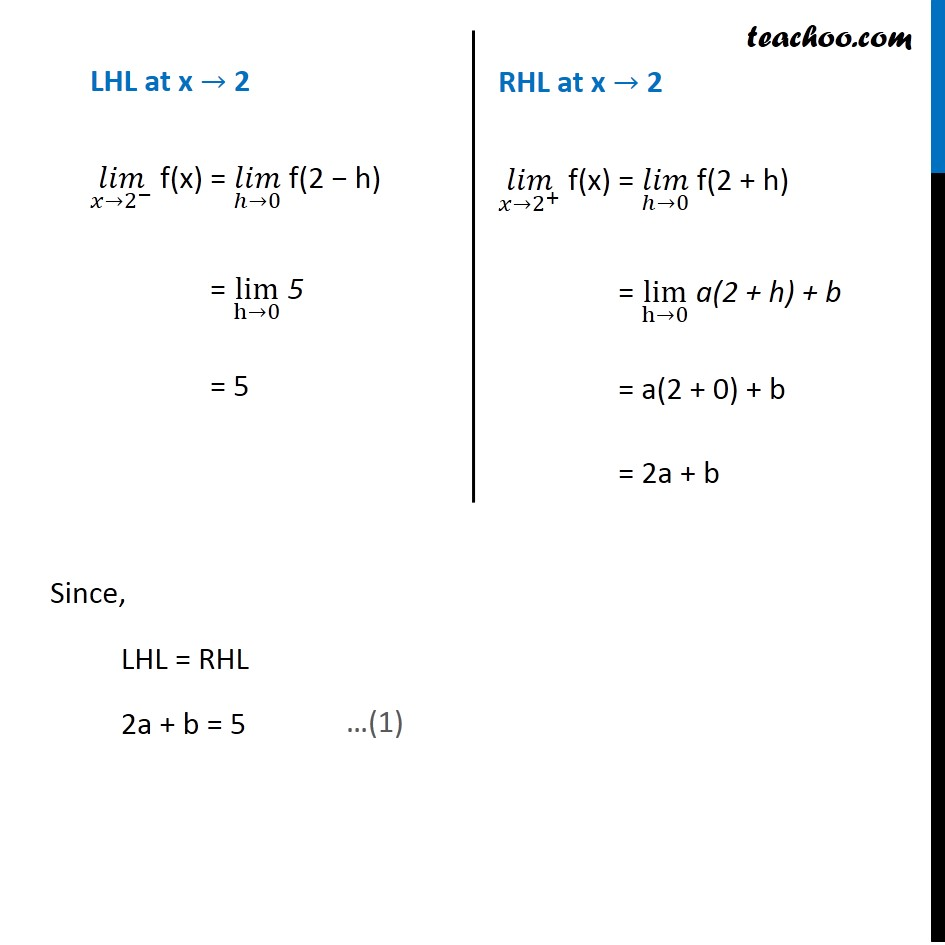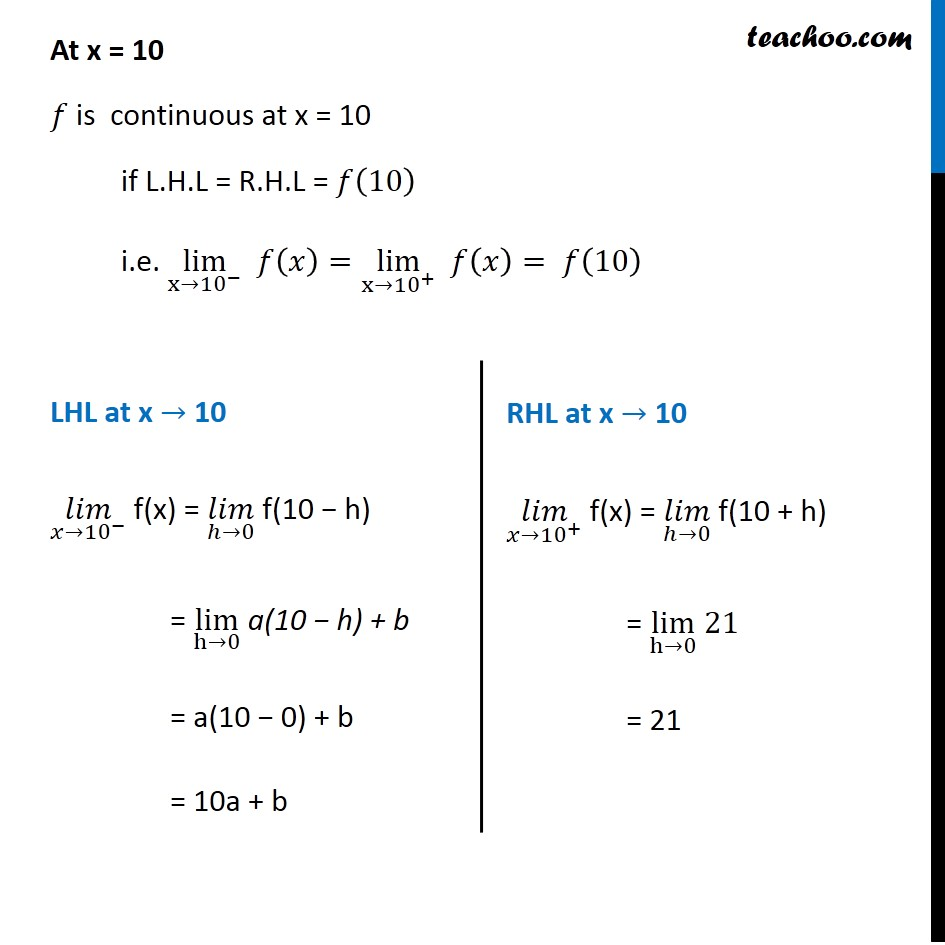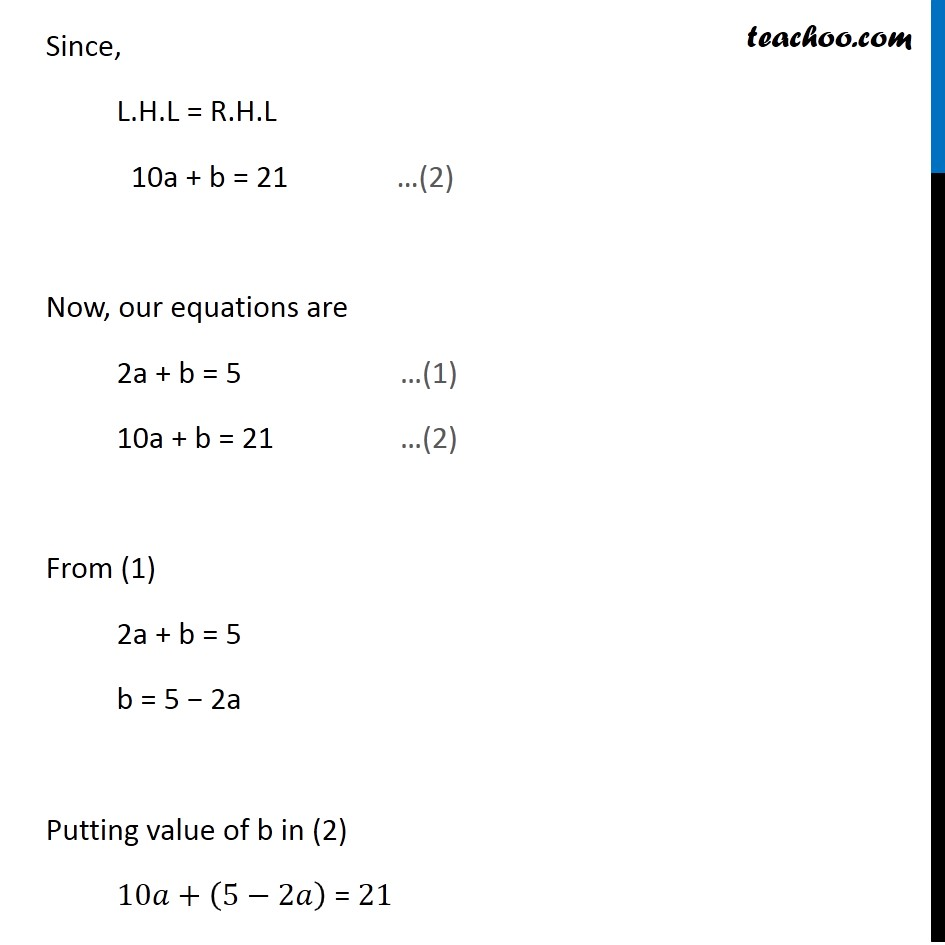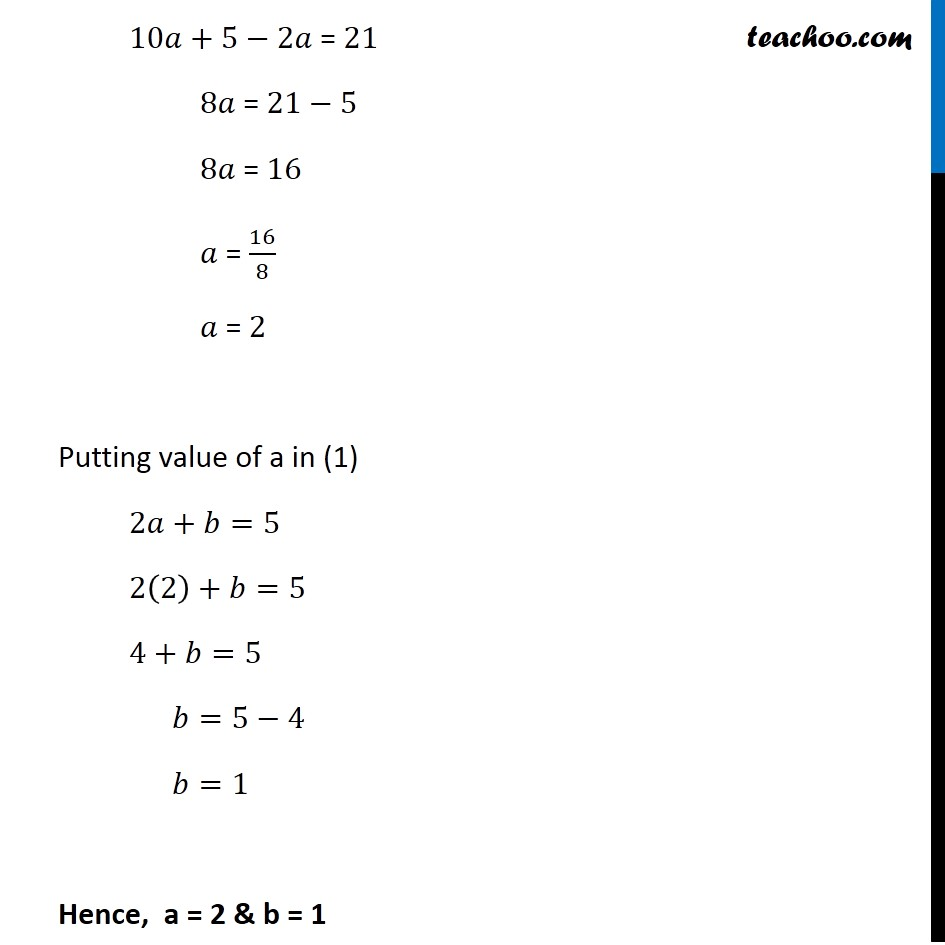1. Chapter 5 Class 12 Continuity and Differentiability
2. Serial order wise
3. Ex 5.1

Transcript

Ex 5.1, 30 Find the values of a and b such that the function defined by 𝑓(𝑥)={█(5, 𝑖𝑓 𝑥≤2@𝑎𝑥+𝑏, 𝑖𝑓 2<𝑥<10@21, 𝑖𝑓 𝑥≥10)┤ is a continuous function Since f(x) is a continuous function, It will be continuous for all values of At x = 2 A function is continuous at x = 2 if L.H.L = R.H.L = 𝑓(2) i.e. lim┬(x→2^− ) 𝑓(𝑥)=lim┬(x→2^+ ) " " 𝑓(𝑥)= 𝑓(2) LHL at x → 2 (𝑙𝑖𝑚)┬(𝑥→2^− ) f(x) = (𝑙𝑖𝑚)┬(ℎ→0) f(2 − h) = lim┬(h→0) 5 = 5 RHL at x → 2 (𝑙𝑖𝑚)┬(𝑥→2^+ ) f(x) = (𝑙𝑖𝑚)┬(ℎ→0) f(2 + h) = lim┬(h→0) a(2 + h) + b = a(2 + 0) + b = 2a + b Since, LHL = RHL 2a + b = 5 At x = 10 𝑓 is continuous at x = 10 if L.H.L = R.H.L = 𝑓(10) i.e. lim┬(x→〖10〗^− ) 𝑓(𝑥)=lim┬(x→〖10〗^+ ) " " 𝑓(𝑥)= 𝑓(10) LHL at x → 10 (𝑙𝑖𝑚)┬(𝑥→〖10〗^− ) f(x) = (𝑙𝑖𝑚)┬(ℎ→0) f(10 − h) = lim┬(h→0) a(10 − h) + b = a(10 − 0) + b = 10a + b RHL at x → 10 (𝑙𝑖𝑚)┬(𝑥→〖10〗^+ ) f(x) = (𝑙𝑖𝑚)┬(ℎ→0) f(10 + h) = lim┬(h→0) 21 = 21 Since, L.H.L = R.H.L 10a + b = 21 Now, our equations are 2a + b = 5 …(1) 10a + b = 21 …(2) From (1) 2a + b = 5 b = 5 − 2a Putting value of b in (2) 10𝑎+(5−2𝑎) = 21 10𝑎+5−2𝑎 = 21 8𝑎 = 21−5 8𝑎 = 16 𝑎 = 16/8 𝑎 = 2 Putting value of a in (1) 2𝑎+𝑏=5 2(2)+𝑏=5 4+𝑏=5 𝑏=5−4 𝑏=1 Hence, a = 2 & b = 1

Ex 5.1